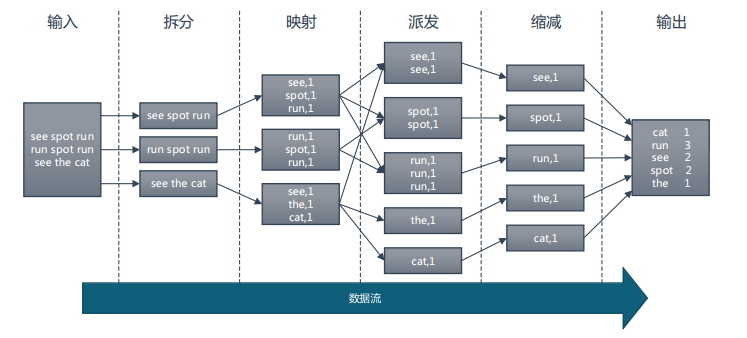2017/04/24 18:17

Mapreduce 计算框架

1) MapReduce 编程模型
2) MapReduce 执行流程
3) MapReduce 数据本地化
4) MapReduce 工作原理
5) MapReduce 错误处理机制

## 1. MapReduce 编程模型

Map和Reduce的概念是从函数式变成语言中借来的，整个MapReduce计算过程分为 Map 阶段和 Reduce阶段， 也称为映射和缩减阶段， 这两个独立的阶段实际上是两个独立的过程，即 Map 过程和 Reduce 过程，在 Map 中进行数据的读取和预处理，之后将预处理的结果发送到 Reduce 中进行合并。

1) 创建 map.py 文件，写入以下代码：

``````#!/usr/bin/env python
import sys
word_list = []
for line in sys.stdin:
word_list = line.strip().split(' ')
if len(word_list) <= 0:
continue
for word in word_list:
w = word.strip()
if len(w) <= 0:
continue
print '\t'.join([w, "1"])``````

``````#!/usr/bin/env python
import sys
cur_word = None
sum_of_word = 0

for line in sys.stdin:
ss = line.strip().split('\t')
if len(ss) != 2:
continue
word = ss.strip()
count = ss.strip()

if cur_word == None:
cur_word = word
if cur_word != word:
print '\t'.join([cur_word, str(sum_of_word)])

sum_of_word = 0
cur_word = word
sum_of_word += int(count)

print '\t'.join([cur_word, str(sum_of_word)])
sum_of_word = 0``````

cat big.txt | python map.py | sort -k1 | python reduce.py2. MapReduce 执行流程

0
0 收藏

### 作者的其它热门文章0 评论
0 收藏
0